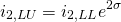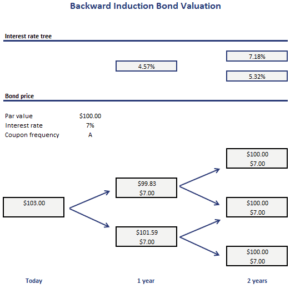# Backward Induction Bond Valuation

Backward Induction bond valuation is a method to value a bond using a binomial interest rate tree. The method starts at the final nodes, that is the point in time where the investor receives principal and the final coupon payment. From these final nodes, we calculate “backwards” the value of the bond at earlier nodes.

It is important to keep in mind that in a binomial interest rate tree model the probability of a down move and an up move is always 50%. This is the case because the interest rates in the interest rate tree are calculated using a specific formula.

On this page, we discuss the backward induction method for bond valuation in more detail. We discuss the backward induction valuation formulas and discuss an example. The example is implemented using an Excel spreadsheet. The calculator can be downloaded at the bottom of the page.

## Backward induction valuation

As we mentioned in the introduction, backward induction is always applied using an interest rate tree that is calculated using a binomial tree. In a binomial tree, the probabilities of an up or down move are always 50%. The one period forward rates in a binomial tree are calculated as followsThis formula states that the forward interest rate for a down move (L) followed by an up move (U) is equal to the interest rate after two down moves (LL) * e^(2*sigma). This means that interest rates in the interest rate tree are two standard deviations apart. If this does not make sense to yet, don’t worry. We will implement the approach in Excel.

As a consequence of the way interest rates in the tree are determined, the value of a bond at a given node is always the average of the present value of the two possible bond values from the next period. The discount rate we should use to calculate the present value is always the forward rate reported in the interest rate tree.

## Backward induction bond valuation example

Let’s turn to an example. The following table presents a interest rate tree and uses the backward induction bond valuation approach using Excel. The spreadsheet is available for download at the bottom of the page. That way, it is possible to have a look at the necessary formulas.## Summary

We discussed the backward induction method to value a bond. The approach is very simple and can easily be applied using a spreadsheet.